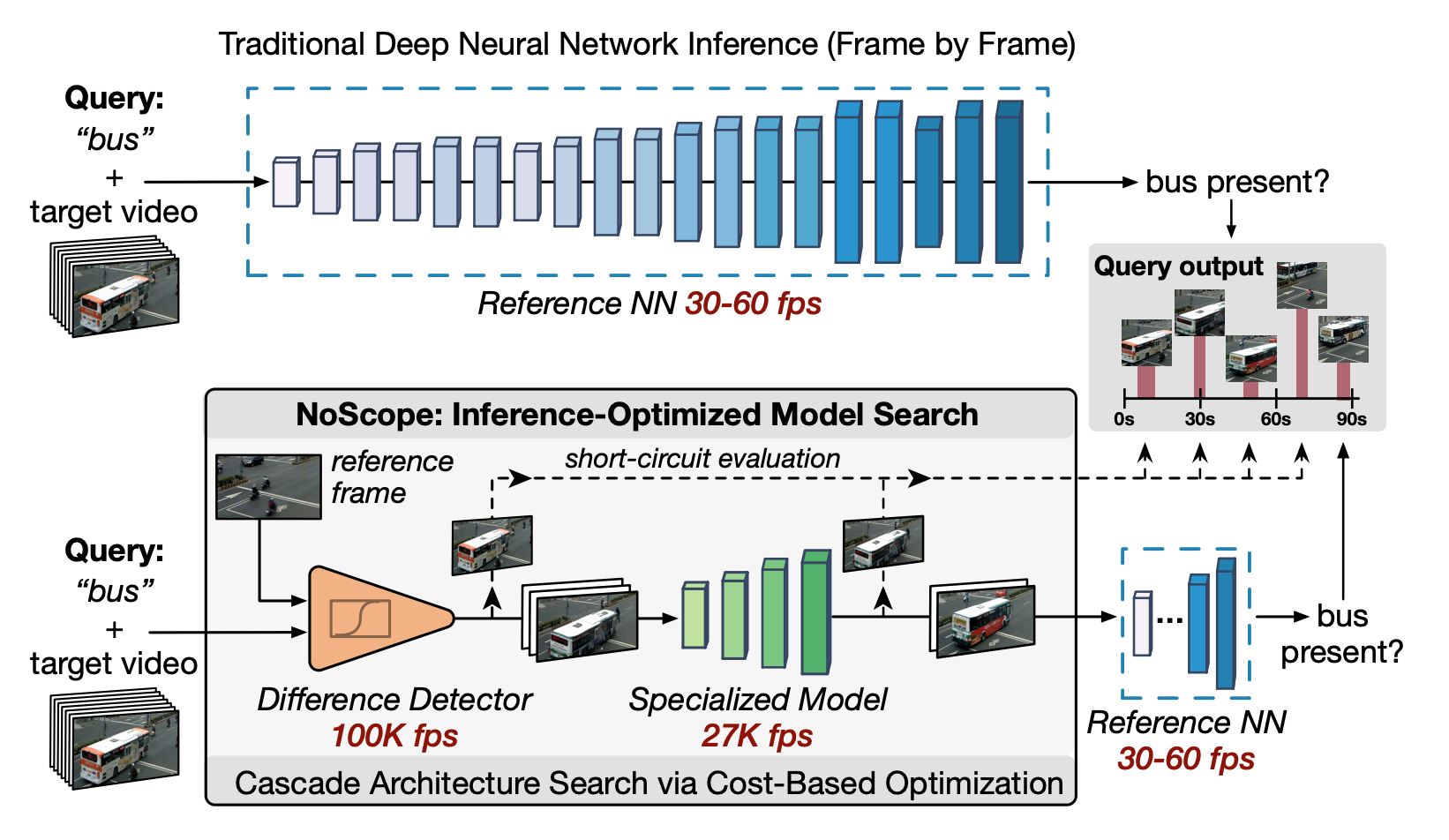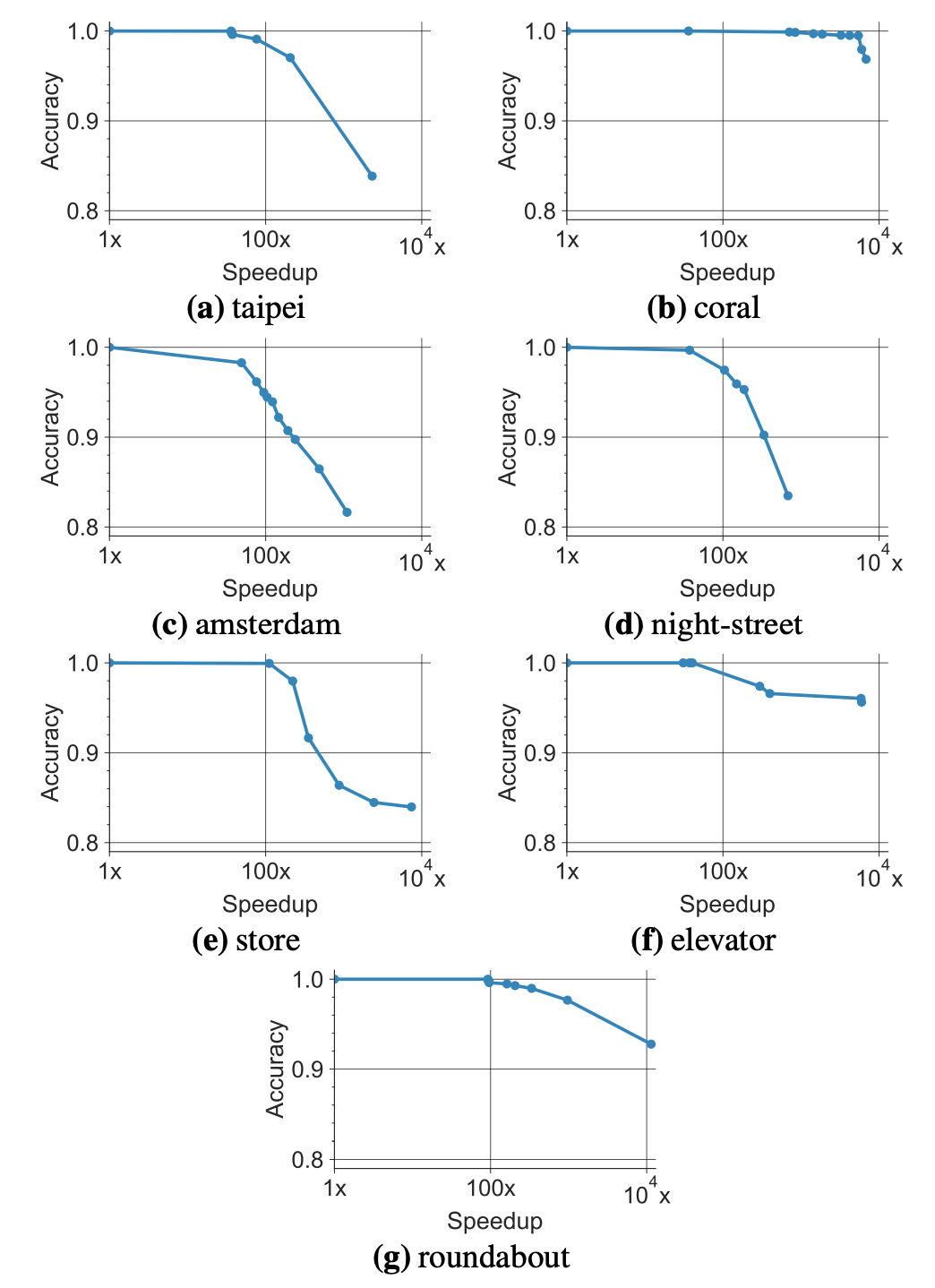# NoScope: Optimizing Neural Network Queries over Video at Scale

01 Nov 2021

NoScope: Optimizing Neural Network Queries over Video at Scale, Daniel Kang et al., VLDB 2017

This is a paper that I like very much and have read multiple times. It introduces to me a new concept of efficient deep learning inferences that I think is highly underrated. It provides an example that in some application scenarios, you can build a cascade of models before using the deep learning model to infer the predictions of an input. The use case the paper introduces is real-time bus detection in videos from fixed-angled cameras. The task is a binary classification (yes/no for buses in the current frame). Every frame has the same background. And the goal is to maximize the throughput of classification results without losing accuracies. Here is what the whole pipeline looks likeThe whole “cascade” can be understood in the following way. The input is first passed into a difference detector (fastest). It tells you whether the current video frame is similar to the frames in the previous seconds. If so, the model would just return the same predictions as the previous frames. If not, the input is passed into a small and lightweight neural network (a specialized model) that is trained based on the original model. If this small model returns a prediction with high confidence, we can return the predictions as well. If not, we would just take the input to the original model to obtain the predictions. Such a pipeline of “IF-ELSE” decisions allows lots of inputs to skip the actual model inferences, thus increasing the throughput of the whole process.

The question now is how to generate the difference detector and the specialized model. For the difference detector, since the camera angle is fixed, we can extract a background image by averaging all the pixels of video frames without cars (which are determined by the original model by passing a set of frames to it). Such a background image can be used as a reference to subtract the “background” part of the frames. Then the residuals would be something meaningful to be compared. The Mean Square Error between the residual frame from the current time and the one in the previous seconds will be the differences. If the difference is small, there is no need to recompute predictions.

For the specialized model, the goal is to make a neural network that is faster with reasonable accuracies. Thus, we can set a lower bound for accuracy, and within the bound, we try to maximize the throughput. An interesting note is that we do not simply go for the simplest architecture for the specialized model to satisfy this objective. It is because if the specialized model is super fast but not accurate at all, the accuracy of the whole pipeline can still be maintained, bypassing all the inputs to the original model. Therefore, the throughput of different architecture is determined by the fractions of the inputs that are passed to different components: $$f_sT_{MSE} + f_sf_mT_{SpecializedNN} + f_sf_mf_cT_{FullNN}$$, where $$f_s,f_m,f_c$$ correspond to the fraction of the inputs passing the difference detector, specialized model, and original model respectively, and $$T$$ are the inference time. Therefore, the search of different architecture can be seen as a Cost-Based Optimizer (CBO) in choosing different “walk plans” of the model setup.

Some experiments on different datasets show that the speedup can be 100$$\times$$ without degrading the accuracies by a lot (all still above 90%).